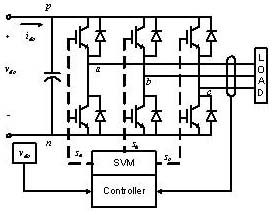LIBRARY

# Predicting Low Frequency Harmonics in Voltage Source Inverter (VSI) Enhanced Average ModelFig. 1. Switching model diagram.
An enhanced average model of a voltage source inverter (VSI) that can accurately predict some of the low frequency phenomena only seen by the switching models is developed. These phenomena include the dead-time, voltage drop across switching devices (switch and diode), different modulation techniques and minimum pulse width. In addition, the effect of inductor current ripple is analyzed, enhancing the accuracy near the zero crossings. Simulation and experimental results with a 2kW prototype are used for validation purposes. The simulation results of both complete switching and proposed average model shown in fig. 1 and 2 respectively are compared depicting less than 0.5% difference in time domain and also harmonic spectrum analysis.

Fig. 3 shows the results comparing the experimental results averaged over one line period with the average model with distortion added: 5µs dead time, on and off time of 1µs, voltage drop across the switch of 1.2V, diode on voltage of 0.7V and 1µs minimum pulse width.Fig. 2. Average model diagram.Fig. 3. Experimental averaged results compared to the enhanced average model.